## IP address Classes- Introduction and Explanation

An IP address is an identifying number for network devices. It is similar to a home or business address supplying that specific physical location with a particular address. Devices on a network differentiated from one another through IP addresses. Having an IP address allows a device to communicate with other devices over an IP-based network just like the internet. The IP addresses are two main standards know as IPv4 and IPv6. In this article, I am going to discuss the IPv4 address. The IPv6 address will be discussed in later articles.

## IPv4 Addressing

IPv4 is the fourth revision of the IP and a widely used protocol for data communication over kinds of networks. It is defined and specified in IETF publication RCF 791. It is a connectionless protocol provides the logical connection between network devices and also providing unique identification for each device. We can configure the IPv4 addresses on the network devices manually as well as automatically – depending on the network type. IPv4 uses 32-bit addresses for Ethernet communication in five types of IP address classes, which is A, B, C, D and E.

## Address Architecture

TCP/IP was introduced in the 1980s, at that time internet uses a two-level addressing scheme. The scheme offered sufficient scalability during the time. The 32-bit-long IPv4 address identifies a network number and a host number, 16 bits reserved for network and 16 bits reserved for host identification, Figure below illustrates the 32-bit address architecture in the 1980s.

##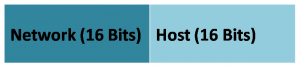The current IPv4 address has also 32 bit long and contains two portions, a network portion, and a host portion. Network portion identifies the network of the host and host portion show the number of the host in the network. Host portion identifies the device or the host. The router uses the network portion of the IP addresses to make routing decisions and to facilitate communication between hosts that belong to different networks. Like routers and computer, humans can’t work with strings of 32 bits (1s and 0s).

Therefore, we express the 32 bit IP address in dotted-decimal notation. As we know that 32-bit address further divided into four groups of eight, called octets. An octet is ranging from 000000000 to 11111111. Each octet converted to decimal and then separated by decimal points or dots. The range of each octet in decimal is from 0 to 255.  For example, 10.10.10.1, 172.16.16.254 and 192.168.0.254 are IP addresses expressed in dotted decimal notations. If we can convert these addresses to binary. For example binary of the above addresses is 00001010. 00001010. 00001010.00000001, 10101100. 00010000.00010000. 11111110, 11000000. 10101000.00000000.11111110.

## IP Address Classes

In the starting of TCP/IP, a class of IP addresses used to define the network and host portions. IPv4 addresses divided into five different IP address classes. The first few bits of the first octet define the class of IP address. These bits are known as high order bits (HOB). Although the class system can still be applied to IP addresses, networks today often ignore the rules of class in favour of a classless IP scheme. The five different IP address classes are A, B, C, D and E.

Class A, B, and C have a different bit length for addressing the network host. Class D addresses reserved for multicasting, while Class E addresses reserved for future use. Each of the four octets of an IP address represents the network portion of the host portion, depending on the address class. The figure below illustrates the network and host portions of the respective Class A, B, C, and D addresses.

##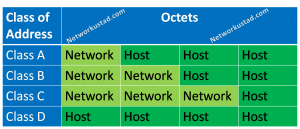The first three IP address classes A, B, and C are actually hosting addresses in any IP networks. But the Class D addresses are used for multicasting. Class E addresses reserved for experiments in labs and medical equipment and are not shown in the above figure.

It is not easy to identify the network and the host portion of any dotted decimal IP address because the IP addresses are not actually four numbers. It containing 32 different numbers or 32 bits (0 or 1s). the figure below illustrates all the important figures of IP address classes.

## Class A

The most significant bit of the class A network is 0, also known as high order bit(HOB). The remaining seven bits in the first octet define the number of networks in class A addresses and the three octets (24 bits) define the number of total IP address available in the class. The figure below illustrates Class A, address bit distribution.

If the first bit of the first octet of an IP address is a binary 0, the address is a Class A address. With the first bit being 0, the lowest number is 00000000 (decimal 0) and the highest number is 01111111 (decimal 127). Any address starts with a value between 0 -127  in the first octet is a Class A address. The numbers, 0 and 127, reserved and cannot be used as a network address.

Class A network can accommodate a very large number of hosts because only first octet reserved for the network number. The three octets or 24 bits reserved for the host portion of the network. Each network of this class supports a maximum of 16,777,214 (2 24 -2) hosts per network. Figure two subtracted because the first and last addresses are reserved as network ID and broadcast IP. The lowest address is 24, 0s in the host portion and the highest address is 24, 1s in the host portion.

##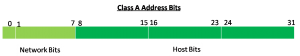The routing table uses network ID for the routing table. So address that has all 0s in the host portion cannot be used with the individual host. For example, 10.0.0.0 is a Class A Address containing all 0s in the host portion. So it is network identification and cannot be configured on network hosts and devices. Every network also requires a broadcast address that can be used to send a message to each host on a network. This address has all 1s on the host portion. For example, a broadcast address for network 10.0.0.0 would be 10.255.255.255.

The total number of the network in Class A is 126. Each of the 126 Class A network has almost 17 million host addresses that makeup 50 % of the entire IPv4 address space. 0.0.0.0 address also reserved for the default route. 127.0.0.0 reserved for the local loopback address, which is why Class A network range is from 1 – 126 and Class B addresses start from the address of 128.0.0.0.

A Class A network provides many possibilities for any organization or a company. Class A network provides a huge global network with those many hosts, the hosts in such a network could not function as members of the same logical group.

The class A addresses create a large broadcast domain but network administrators need smaller logical groupings to control broadcasts, apply policies, and troubleshoot problems. This is possible by applying a subnet mask which allows subnetting. A subnetting breaks a large block of addresses into smaller groups called subnetworks. Subnetting avoids waste of IP address and it’s also helpful for better efficiency. Default subnet mask for a class A IP address is 255.0.0.0. Private IP address range for class A is 10.0.0.0 to 10.255.255.255.

## Class B

The high order bit of class B addresses is starts with a binary 10 in the first 2 bits of the first octet. So, the lowest number of class B address is 10000000 (decimal 128) and the highest number is 10111111 (decimal 191). So any IP address starts with a range between 128 – 191 in the first octet is class B IP address. Class B network can provide addressing scheme to medium-size networks.

In Class B, the first two octets represent the network, and the other two octets represent the host portion of the address. So class B has 16384 (214) Network addresses and 65534 (216-2) Host addresses. So, each Class B network can support up to 65,534 hosts. The class B network is much smaller than the networks of Class A. Just like Class A networks, we can subnet the class B network to improve network efficiency. The figure below illustrates 32 bits distribution of Class B network.

##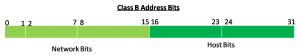The first octet of a Class B address has 64 possibilities that are from 128 to 191. The second octet has 256 possibilities that are from 0 to 255. That gives 16,384 networks. We can simply multiply 64 with 256 that will total possible network in class B. Default subnet mask for class B IP addresses is 255.255.0.0. Class B addresses also a private IP address range. Which is from 172.16.0.0 to 172.31.255.255.

## Class C

The high order bits of class C address is binary 110. So, the lowest number that starts with binary 110 is 11000000 (Decimal 192) and the highest number that starts with binary 110 is 11011111 (Decimal 223). So the range of class C is from 192 to 223 in the first octet. Class C addresses initially planned for a small network. The first three octets of a Class C address represent the network portion of the IP address. The fourth octet represents the host portion. The figure below illustrates class C address bits distribution.

##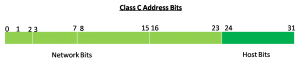As we learn that the first 3 bits of a Class C address are always 110, So 21 bits are left in the network portion of the address, which results in 221 or 2,097,152 Class C networks. Each network containing a 254 (28 -2) hosts each. The first and last addresses are reserved for network identification and broadcasting. Default subnet mask for class C IP addresses is 255.255.255.0. Class C addresses are also a private IP address range starting from 192.168.0.0 to 192.168.255.255

## Class D

The high order bit of  Class D address is 1110 in the first octet. So, the lowest number starting with 1110 is binary 11100000 (Decimal 224) and the highest number is 11101111 (Decimal 239). So the range of class D addresses is from 224-239 in the first octet. Class D addresses not used with individual host, it can be used with a group of hosts called a host group or multicast group.

It is used when a single message required sending to many selected recipients known as multicast or multicasting. Multicast is different from the broadcast. Every device on a logical network must process a broadcast, whereas only devices configured to listen for a Class D address receive a multicast.

## Class E

The high order bits of class E IP address begins with 1111, the range of this class is starting with 11110000 (decimal 240) and end with 11111111 (decimal 255). So the range of class E is from 240 to 255 in the first octet. Class E addresses reserved for experimental purposes.